# Algorithm

Detailed instructions defining a computational process (which is then said to be algorithmic), which begins with an arbitrary input (out of a certain number of inputs which are possible for the given algorithm), and with instructions aimed at obtaining a result (or output) which is fully determined by the input. For instance, the rules taught in elementary schools for column-wise addition, subtraction, multiplication and division are algorithms; in these algorithms the possible results are non-negative integers written in the decimal system, while the possible inputs are ordered pairs of such numbers. It is, in general, not assumed that the result is necessarily obtained: the process of applying an algorithm to some possible input (i.e. an algorithmic process which proceeds starting from this input) may also terminate without a result being obtained (in such a case one has a no-result stop) or may not terminate at all. If the process terminates (does not terminate), then one says that the input is suitable (is not suitable) for the algorithm in question.

An important result in this area is the undecidability of the so-called halting problem. It is possible to construct an algorithmsuch that there is no algorithm to decide whether or not some arbitrary input which is possible foris also suitable for. In particular, one can find such an algorithmfor which the set of its possible inputs is the set of natural numbers.

The notion of an algorithm is one of the central concepts in modern mathematics, especially in the area of computations. An example is given below. Finding the numerical solution of equations of a given type is tantamount to constructing an algorithm which converts an arbitrary equation of this type and an arbitrary positive rational numberinto a number (or a set of numbers) which differs from the root(s) of this equation by less than. However, the term "computational process" , as used in the context of an algorithm, must not be understood to mean merely numerical calculations: even in elementary algebra computations with letters are used. Standard arithmetic calculations involve some symbols which are not numbers (parentheses, equality signs, symbols for arithmetic operators). It is accordingly expedient to consider algorithms involving arbitrary symbols and objects composed from symbols. The simplest example of such an object is a linear sequence of symbols forming a word. It is also possible to consider "non-linear" objects — algebraic matrices, derivation trees of some formal language and general graphs. Intuitively, the least requirement on inputs and results of algorithms is that they must be constructive objects (cf. Constructive object). Thus, the concept of an algorithm is very general. One may speak of an algorithm for the translation from one language into another, of an algorithm of an air traffic controller (processing information on the motions of aircraft according to fixed rules), and other examples of algorithmically described control processes. Consequently, the concept of an algorithm is one of the central ideas in cybernetics and computer science.

## Example of an algorithm.

Let the possible inputs and the possible results be all possible words over the alphabet. The transition from a wordto a word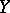is "permissible" in the following two cases (where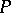denotes an arbitrary word): 1)is of the form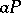, andis of the form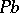; or 2)is of the form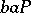, whileis of the form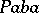. The instructions are formulated as follows: "designating some word as the input, execute the permitted transitions until a word of the form aaP is obtained; then halt and let word P be the result" . These instructions constitute an algorithm, which will be denoted by. Take the word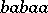as the input. After one transition one obtains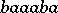; after the second, one obtains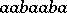. Here the algorithm halts withas result. Consider another input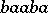. One obtains, in succession,. It can be shown that this process will never end (i.e. none of the generated words will ever begin with). Now take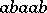as input. One obtains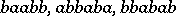; no further permissible transitions are possible, and, at the same time, the termination condition is not satisfied. One has a no-result stop. Thus, inputis suitable for, and inputsandare not.

## The significance of algorithms.

In science, algorithms are encountered everywhere: a "general" solution to a problem requires an algorithm. The ability of man to add numbers is an example of this. Not the fact that he can find, sooner or later, the sum of any two numbers, but rather in the sense that he possesses a method for the unique addition of any two numbers given in written form, i.e. an addition algorithm such as the well-known column-wise addition method. "Generally" solving a problem is formalized as solving an algorithmic problem. An algorithmic problem consists of separate problem instances, and a single algorithm needs to be found for the solution of all of them. If there is no such algorithm, one says that the algorithmic problem is unsolvable. Thus, the problem of numerically solving equations of a given type and the problem of automatic translation are algorithmic problems. Their problem instances are, respectively, to find the numerical solution of individual equations of the given type, and the translation of individual phrases. Other examples are the verification of algebraic equalities in algebra; the algorithmic problem of recognition of the deducibility of propositions from given axioms in mathematical logic. (In mathematical logic the concept of an algorithm is also important since it serves as the basis of the key concept of a calculus, which is a generalization and a more precise form of the intuitive concepts of "proof" and "demonstration" .) The establishment of the unsolvability of a given algorithmic problem (e.g. the problem of establishing the truth or demonstrability of sentences in some logical-mathematical language) is important, because it shows that, in principle, specific problem instances of that problem can only be solved by methods which are specific to each individual problem instance.

The scientific importance of the concept of an algorithm has been recognized for a long time. Since the earliest times, people have looked for constructive methods to solve mathematical problems. Such efforts usually benefitted from the new availability of suitable symbolic notations. This process has made a significant contribution to scientific knowledge, especially so after it had become clear that some problems are inherently unsolvable (squaring the circle, etc.). After it had been recognized that certain problems cannot be solved by direct calculations, the theoretical concept of a set was born in the 19th century. It is only after a period of rapid development of this concept (which did not involve problems of constructive methods in their modern sense), that it was possible in the mid-20th century to return to constructive problems, but this was done on a different level, enriched by the concept of an algorithm which had crystallized in the meantime. This concept forms the base of the constructive trend in mathematics.

The word "algorithm" comes from the word "algoritmi" , the latter being the Latin transliteration of the name of the 9th century Arab mathematician Al-Khwarizmi. An "algorithm" in the Europe of the Middle Ages was "the decimal-positional system and the art of calculating with it" , since it is through the Latin translation (12th century) of the treatise of Al-Khwarizmi that the positional system was introduced in Europe.

## The structure of an algorithmic process.

An algorithmic process is a process of consecutive conversion of constructive objects by discrete "steps" , each step consisting of the replacement of one constructive object by another one. Thus, when applying the algorithmto the word, there follow, in succession, the words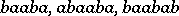, etc. If one applies, say, the algorithm of column-wise subtraction to the pair of numbers <307, 49 >, then one obtains, successively, the following constructive objects:It will be noted that, in a series of successive constructive objects, each successive constructive object is fully determined (in the framework of the given algorithm) by the constructive object immediately preceding it. In the more rigorous approach, it is also assumed that the transition from any constructive object to the one immediately following it is sufficiently "elementary" — in the sense that the one-step transformation of the preceding into the immediately succeeding object is local (it is not the entire constructive object which is transformed, but only its algorithm-limited part; moreover, this transformation itself is not determined by the complete preceding object, but only by a limited part of it).

Accordingly, one has not only a set of possible inputs and a set of possible results, but also a set of possible intermediate results, representing the working medium in which the algorithmic process is evolving. Regarding, all these three sets coincide, but this is not true of the algorithm of column-wise subtraction — the possible inputs are pairs of numbers, the possible results are numbers (all in the decimal system), while the possible intermediate results are "three-storey" records of the type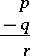where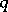is the written record of the number in the decimal system,is a similar record or the empty word, whileis the written record of a number in the decimal system, with dots over some of the digits. As a rule, several (not independent) parameters which are characteristic of an algorithm may be distinguished: 1) the set of possible inputs; 2) the set of possible results; 3) the set of possible intermediate results; 4) the starting rule; 5) the direct transition rule; 6) the termination rule; and 7) the rule for the retrieval of the result.

## Formalizing the concept of an algorithm.

The concept of an algorithm in its general form is a fundamental mathematical concept which cannot be defined in terms of simpler concepts. Strictly speaking, formalizations of the concept of an algorithm actually restrict it somewhat. In each such formalization an exact definition is given of some class within which each one of the parameters may vary. The various formalizations differ from each other by the selection of such classes. Since the parameters unambiguously define some algorithm, a choice of parameter domains determines some class of algorithms. However, such a choice may claim to be a formalization of the intuitive concept of algorithm, only if one can be sure that for any "intuitive" algorithm there exists an equivalent algorithm in the class of algorithms defined by the choice under consideration. This requirement is formulated for each formalization as a fundamental hypothesis, which, in the present state of knowledge, cannot be mathematically demonstrated.

The first formalizations of this type are due to E.L. Post

and to A.M. Turing , , whose constructions anticipated in many respects the ideas on which modern computers are based. There are also versions formulated by A.A. Markov ,

(cf. Normal algorithm) and by A.N. Kolmogorov , , who based the approach on constructive topological complexes of a certain type, which makes it possible to express more precisely the property of "being local" of a transformation. In each of these formalizations the fundamental hypothesis is in satisfactory agreement with practice. Another argument in favour of this hypothesis is the fact that, as can be demonstrated, all the formal versions (of effective computability) which have been proposed are equivalent.

An an example, consider the formalization proposed by Turing. In its original form, this was a description of some theoretical computer consisting of 1) an infinite tape, divided into consecutive cells, each cell capable of containing some symbol out of the "tape alphabet" of the machine; and 2) a "finite control," which at each moment of time is in some "state" (out of a given finite list of states). The finite control can move along the tape, one cell at a time, and change the contents of the cells it passes. A program which monitors the motion of the finite control constitutes the algorithm for the computations conducted on such a machine (a "Turing algorithm" ). For a more exact and more detailed description see Turing machine. Here a modernized exposition of Turing's construction is given. To define a Turing algorithm, one specifies a) pairwise disjoint alphabets, with a distinguished letterinand distinguished lettersandin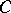; and b) a set of pairs of the form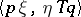where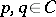,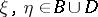, whileis one of the three symbols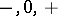; in this set (which is called a program) there are no two pairs with the same first coordinate. The possible inputs and the possible outputs are words over, while the possible intermediate results are words over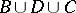which contain not more than one letter of. The starting rule is that the initial wordis converted to the word. The termination rule is that an intermediate result which containsis final. The rule for the retrieval of the output is that the output is the sequence of letters in the final intermediate result which followsand precedes the first letter not belonging to. The transformation rule which convertsinto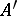is as follows. The letteris written to the left and to the right of; in the word thus formed, the part of the form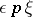, where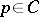,, is replaced by a word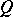in accordance with the rule: a pair with the first termis sought in the program; let the second term of this pair be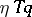; ifis,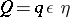; if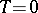,; ifis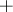,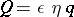. The word produced by this exchange is. For references, see , , , , , ,